# Python Feature Engineering Cookbook3.8 (4 reviews total)
• \$28.99 eBook
• \$41.99 Print + eBook
• \$12.99 eBook + Subscription
What do you get with a Packt Subscription?

• Constantly updated with 100+ new titles each month
• Breadth and depth in over 1,000+ technologies

Feature engineering is invaluable for developing and enriching your machine learning models. In this cookbook, you will work with the best tools to streamline your feature engineering pipelines and techniques and simplify and improve the quality of your code.

Using Python libraries such as pandas, scikit-learn, Featuretools, and Feature-engine, you’ll learn how to work with both continuous and discrete datasets and be able to transform features from unstructured datasets. You will develop the skills necessary to select the best features as well as the most suitable extraction techniques. This book will cover Python recipes that will help you automate feature engineering to simplify complex processes. You’ll also get to grips with different feature engineering strategies, such as the box-cox transform, power transform, and log transform across machine learning, reinforcement learning, and natural language processing (NLP) domains.

By the end of this book, you’ll have discovered tips and practical solutions to all of your feature engineering problems.

Publication date:
January 2020
Publisher
Packt
Pages
372
ISBN
9781789806311

# Foreseeing Variable Problems When Building ML Models

A variable is a characteristic, number, or quantity that can be measured or counted. Most variables in a dataset are either numerical or categorical. Numerical variables take numbers as values and can be discrete or continuous, whereas for categorical variables, the values are selected from a group of categories, also called labels.

Variables in their original, raw format are not suitable to train machine learning algorithms. In fact, we need to consider many aspects of a variable to build powerful machine learning models. These aspects include variable type, missing data, cardinality and category frequency, variable distribution and its relationship with the target, outliers, and feature magnitude.

Why do we need to consider all these aspects? For multiple reasons. First, scikit-learn, the open source Python library for machine learning, does not support missing values or strings (the categories) as inputs for machine learning algorithms, so we need to convert those values into numbers. Second, the number of missing values or the distributions of the strings in categorical variables (known as cardinality and frequency) may affect model performance or inform the technique we should implement to replace them by numbers. Third, some machine learning algorithms make assumptions about the distributions of the variables and their relationship with the target. Finally, variable distribution, outliers, and feature magnitude may also affect machine learning model performance. Therefore, it is important to understand, identify, and quantify all these aspects of a variable to be able to choose the appropriate feature engineering technique. In this chapter, we will learn how to identify and quantify these variable characteristics.

This chapter will cover the following recipes:

• Identifying numerical and categorical variables
• Quantifying missing data
• Determining cardinality in categorical variables
• Pinpointing rare categories in categorical variables
• Identifying a linear relationship
• Identifying a normal distribution
• Distinguishing variable distribution
• Highlighting outliers
• Comparing feature magnitude

# Technical requirements

Throughout this book, we will use many open source Python libraries for numerical computing. I recommend installing the free Anaconda Python distribution (https://www.anaconda.com/distribution/), which contains most of these packages. To install the Anaconda distribution, follow these steps:

1. Visit the Anaconda website: https://www.anaconda.com/distribution/.
The recipes in this book were written in Python 3.7. However, they should work in Python 3.5 and above. Check that you are using similar or higher versions of the numerical libraries we'll be using, that is, NumPy, pandas, scikit-learn, and others. The versions of these libraries are indicated in the requirement.txt file in the accompanying GitHub repository (https://github.com/PacktPublishing/Python-Feature-Engineering-Cookbook).

In this chapter, we will use pandas, NumPy, Matplotlib, seaborn, SciPy, and scikit-learn. pandas provides high-performance analysis tools. NumPy provides support for large, multi-dimensional arrays and matrices and contains a large collection of mathematical functions to operate over these arrays and over pandas dataframes. Matplotlib and seaborn are the standard libraries for plotting and visualization. SciPy is the standard library for statistics and scientific computing, while scikit-learn is the standard library for machine learning.

To run the recipes in this chapter, I used Jupyter Notebooks since they are great for visualization and data analysis and make it easy to examine the output of each line of code. I recommend that you follow along with Jupyter Notebooks as well, although you can execute the recipes in other interfaces.

The recipe commands can be run using a .py script from a command prompt (such as the Anaconda Prompt or the Mac Terminal) using an IDE such as Spyder or PyCharm or from Jupyter Notebooks, as in the accompanying GitHub

In this chapter, we will use two public datasets: the KDD-CUP-98 dataset and the Car Evaluation dataset. Both of these are available at the UCI Machine Learning Repository.

Dua, D. and Graff, C. (2019). UCI Machine Learning Repository (http://archive.ics.uci.edu/ml). Irvine, CA: University of California, School of Information and Computer Science.

1. Visit the following website: https://archive.ics.uci.edu/ml/machine-learning-databases/kddcup98-mld/epsilon_mirror/.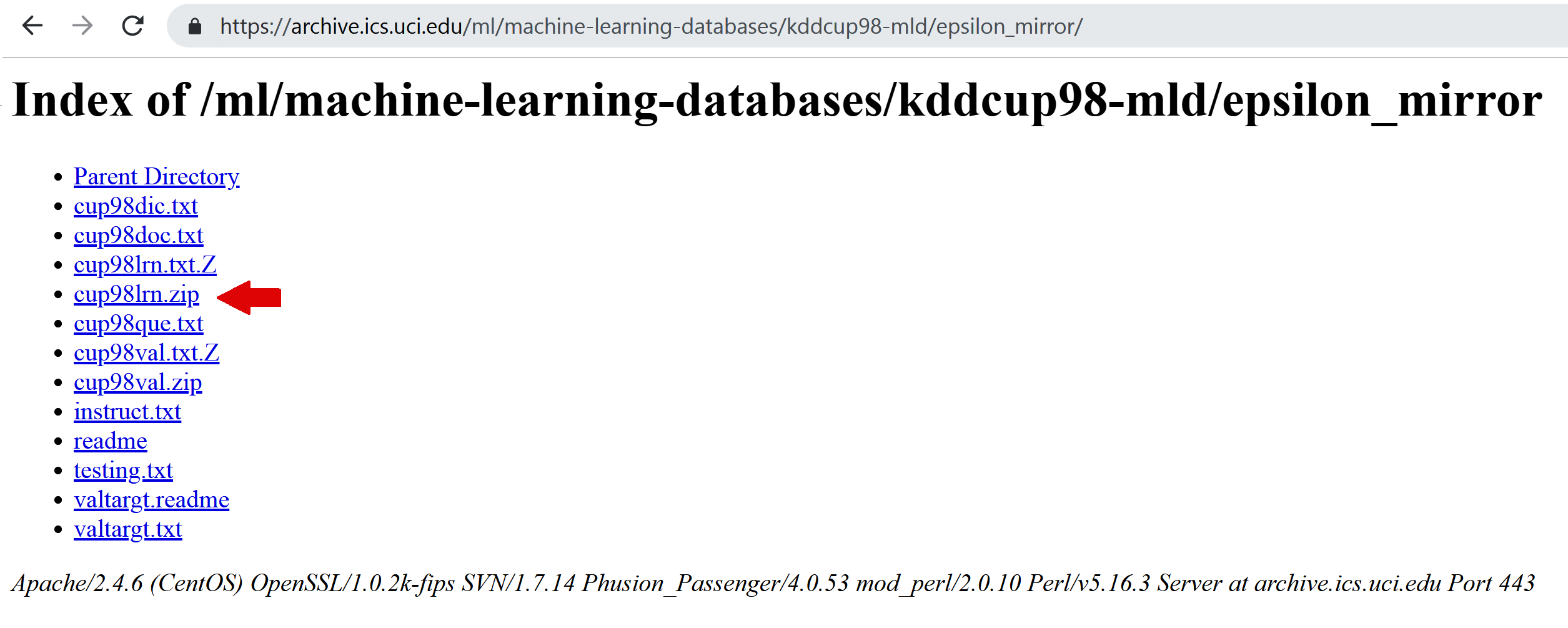1. Unzip the file and save cup98LRN.txt in the same folder where you'll run the commands of the recipes.

1. Go to the UCI website: https://archive.ics.uci.edu/ml/machine-learning-databases/car/.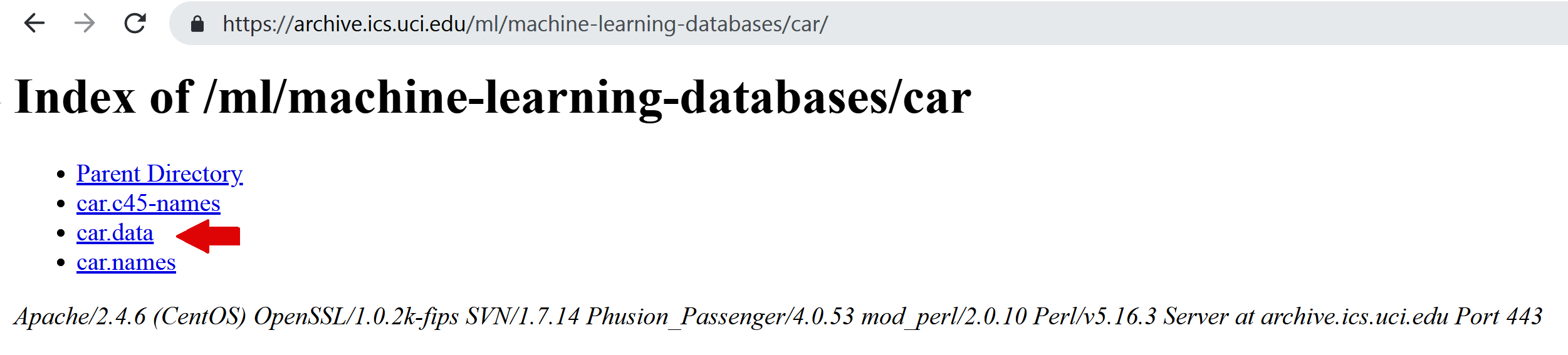1. Save the file in the same folder where you'll run the commands of the recipes.

We will also use the Titanic dataset that's available at http://www.openML.orgTo download and prepare the Titanic dataset, open a Jupyter Notebook and run the following commands:

`import numpy as npimport pandas as pddef get_first_cabin(row):    try:        return row.split()    except:        return np.nan url = "https://www.openml.org/data/get_csv/16826755/phpMYEkMl"data = pd.read_csv(url)data = data.replace('?', np.nan)data['cabin'] = data['cabin'].apply(get_first_cabin)data.to_csv('titanic.csv', index=False)`

The preceding code block will download a copy of the data from http://www.openML.org and store it as a titanic.csv file in the same directory from where you execute the commands.

There is a Jupyter Notebook with instructions on how to download and prepare the titanic dataset in the accompanying GitHub repository: https://github.com/PacktPublishing/Python-Feature-Engineering-Cookbook/blob/master/Chapter01/DataPrep_Titanic.ipynb.

# Identifying numerical and categorical variables

Numerical variables can be discrete or continuous. Discrete variables are those where the pool of possible values is finite and are generally whole numbers, such as 1, 2, and 3. Examples of discrete variables include the number of children, number of pets, or the number of bank accounts. Continuous variables are those whose values may take any number within a range. Examples of continuous variables include the price of a product, income, house price, or interest rate. Categorical variables are values that are selected from a group of categories, also called labels. Examples of categorical variables include gender, which takes values of male and female, or country of birth, which takes values of Argentina, Germany, and so on.

In this recipe, we will learn how to identify continuous, discrete, and categorical variables by inspecting their values and the data type that they are stored and loaded with in pandas.

Discrete variables are usually of the int type, continuous variables are usually of the float type, and categorical variables are usually of the object type when they're stored in pandas. However, discrete variables can also be cast as floats, while numerical variables can be cast as objects. Therefore, to correctly identify variable types, we need to look at the data type and inspect their values as well. Make sure you have the correct library versions installed and that you've downloaded a copy of the Titanic dataset, as described in the Technical requirements section.

# How to do it...

First, let's import the necessary Python libraries:

1. Load the libraries that are required for this recipe:
`import pandas as pdimport matplotlib.pyplot as plt`
1. Load the Titanic dataset and inspect the variable types:
`data = pd.read_csv('titanic.csv')data.dtypes`

The variable types are as follows:

```pclass         int64
survived       int64
name          object
sex           object
age          float64
sibsp          int64
parch          int64
ticket        object
fare         float64
cabin         object
embarked      object
boat          object
body         float64
home.dest     object
dtype: object```
In many datasets, integer variables are cast as float. So, after inspecting the data type of the variable, even if you get float as output, go ahead and check the unique values to make sure that those variables are discrete and not continuous.
1. Inspect the distinct values of the sibsp discrete variable:
`data['sibsp'].unique()`

The possible values that sibsp can take can be seen in the following code:

`array([0, 1, 2, 3, 4, 5, 8], dtype=int64)`
1. Now, let's inspect the first 20 distinct values of the continuous variable fare:
`data['fare'].unique()[0:20]`

The following code block identifies the unique values of fare and displays the first 20:

```array([211.3375, 151.55  ,  26.55  ,  77.9583,   0.    ,  51.4792,
49.5042, 227.525 ,  69.3   ,  78.85  ,  30.    ,  25.925 ,
247.5208,  76.2917,  75.2417,  52.5542, 221.7792,  26.    ,
91.0792, 135.6333])```

Go ahead and inspect the values of the embarked and cabin variables by using the command we used in step 3 and step 4.

The embarked variable contains strings as values, which means it's categorical, whereas cabin contains a mix of letters and numbers, which means it can be classified as a mixed type of variable.

# How it works...

In this recipe, we identified the variable data types of a publicly available dataset by inspecting the data type in which the variables are cast and the distinct values they take. First, we used pandas read_csv() to load the data from a CSV file into a dataframe. Next, we used pandas dtypes to display the data types in which the variables are cast, which can be float for continuous variables, int for integers, and object for strings. We observed that the continuous variable fare was cast as float, the discrete variable sibsp was cast as int, and the categorical variable embarked was cast as an objectFinally, we identified the distinct values of a variable with the unique() method from pandas. We used unique() together with a range, [0:20], to output the first 20 unique values for fare, since this variable shows a lot of distinct values.

# There's more...

To understand whether a variable is continuous or discrete, we can also make a histogram:

1. Let's make a histogram for the sibsp variable by dividing the variable value range into 20 intervals:
`data['sibsp'].hist(bins=20)`

The output of the preceding code is as follows: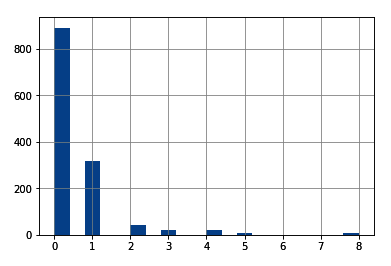Note how the histogram of a discrete variable has a broken, discrete shape.

1. Now, let's make a histogram of the fare variable by sorting the values into 50 contiguous intervals:
`data['fare'].hist(bins=50)`

The output of the preceding code is as follows: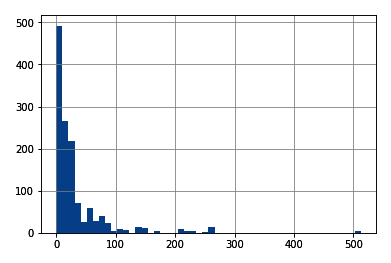The histogram of continuous variables shows values throughout the variable value range.

# Quantifying missing data

Missing data refers to the absence of a value for observations and is a common occurrence in most datasets. Scikit-learn, the open source Python library for machine learning, does not support missing values as input for machine learning models, so we need to convert these values into numbers. To select the missing data imputation technique, it is important to know about the amount of missing information in our variables. In this recipe, we will learn how to identify and quantify missing data using pandas and how to make plots with the percentages of missing data per variable.

In this recipe, we will use the KDD-CUP-98 dataset from the UCI Machine Learning Repository. To download this dataset, follow the instructions in the Technical requirements section of this chapter.

# How to do it...

First, let's import the necessary Python libraries:

1. Import the required Python libraries:
`import pandas as pdimport matplotlib.pyplot as plt`
1. Let's load a few variables from the dataset into a pandas dataframe and inspect the first five rows:
`cols = ['AGE', 'NUMCHLD', 'INCOME', 'WEALTH1', 'MBCRAFT', 'MBGARDEN', 'MBBOOKS', 'MBCOLECT', 'MAGFAML','MAGFEM', 'MAGMALE']`
`data = pd.read_csv('cup98LRN.txt', usecols=cols)data.head()`

After loading the dataset, this is how the output of head() looks like when we run it from a Jupyter Notebook: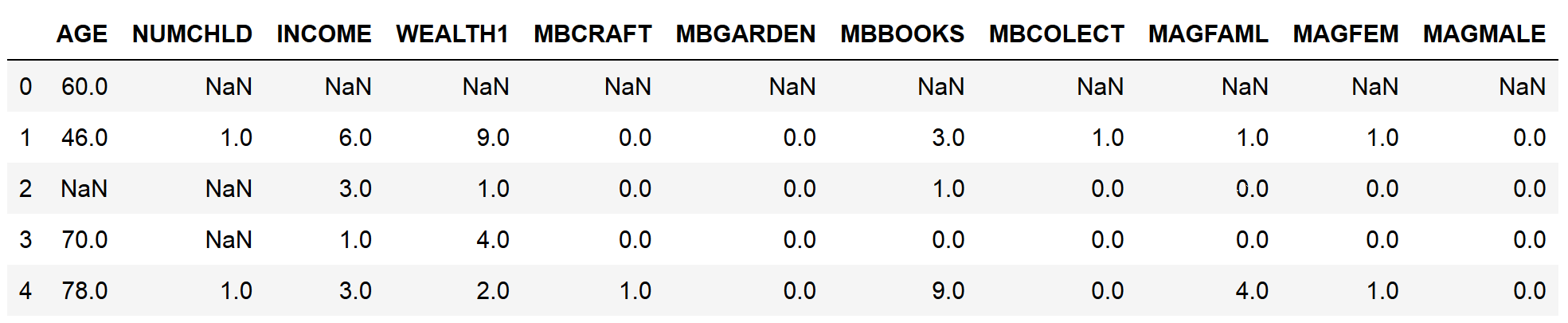1. Let's calculate the number of missing values in each variable:
`data.isnull().sum()`

The number of missing values per variable can be seen in the following output:

```AGE         23665
NUMCHLD     83026
INCOME      21286
WEALTH1     44732
MBCRAFT     52854
MBGARDEN    52854
MBBOOKS     52854
MBCOLECT    52914
MAGFAML     52854
MAGFEM      52854
MAGMALE     52854
dtype: int64```
1. Let's quantify the percentage of missing values in each variable:
`data.isnull().mean()`

The percentages of missing values per variable can be seen in the following output, expressed as decimals:

```AGE         0.248030
NUMCHLD     0.870184
INCOME      0.223096
WEALTH1     0.468830
MBCRAFT     0.553955
MBGARDEN    0.553955
MBBOOKS     0.553955
MBCOLECT    0.554584
MAGFAML     0.553955
MAGFEM      0.553955
MAGMALE 0.553955
dtype: float64```
1. Finally, let's make a bar plot with the percentage of missing values per variable:
`data.isnull().mean().plot.bar(figsize=(12,6))plt.ylabel('Percentage of missing values')plt.xlabel('Variables')plt.title('Quantifying missing data')`

The bar plot that's returned by the preceding code block displays the percentage of missing data per variable: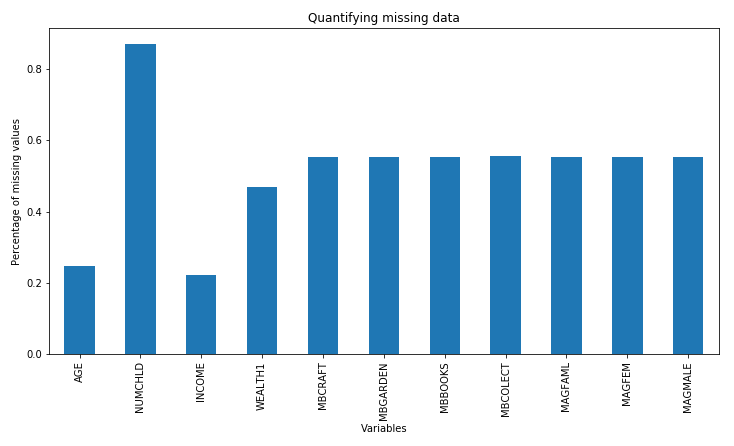We can change the figure size using the figsize argument within pandas plot.bar() and we can add x and y labels and a title with the plt.xlabel(), plt.ylabel(), and plt.title() methods from Matplotlib to enhance the aesthetics of the plot.

# How it works...

In this recipe, we quantified and displayed the amount and percentage of missing data of a publicly available dataset.

To load data from the txt file into a dataframe, we used the pandas read_csv() method. To load only certain columns from the original data, we created a list with the column names and passed this list to the usecols argument of read_csv(). Then, we used the head() method to display the top five rows of the dataframe, along with the variable names and some of their values.

To identify missing observations, we used pandas isnull(). This created a boolean vector per variable, with each vector indicating whether the value was missing (True) or not (False) for each row of the dataset. Then, we used the pandas sum() and mean() methodto operate over these boolean vectors and calculate the total number or the percentage of missing values, respectively. The sum() method sums the True values of the boolean vectors to find the total number of missing values, whereas the mean() method takes the average of these values and returns the percentage of missing data, expressed as decimals.

To display the percentages of the missing values in a bar plot, we used pandas isnull() and mean(), followed by plot.bar(), and modified the plot by adding axis legends and a title with the xlabel(), ylabel(), and title() Matplotlib methods.

# Determining cardinality in categorical variables

The number of unique categories in a variable is called cardinality. For example, the cardinality of the Gender variable, which takes values of female and male, is 2, whereas the cardinality of the Civil status variable, which takes values of married, divorced, singled, and widowed, is 4. In this recipe, we will learn how to quantify and create plots of the cardinality of categorical variables using pandas and Matplotlib.

In this recipe, we will use the KDD-CUP-98 dataset from the UCI Machine Learning Repository. To download this dataset, follow the instructions in the Technical requirements section of this chapter.

# How to do it...

Let's begin by importing the necessary Python libraries:

1. Import the required Python libraries:
`import pandas as pdimport numpy as npimport matplotlib.pyplot as plt`
1. Let's load a few categorical variables from the dataset:
`cols = ['GENDER', 'RFA_2', 'MDMAUD_A', 'RFA_2', 'DOMAIN', 'RFA_15']data = pd.read_csv('cup98LRN.txt', usecols=cols)`
1.  Let's replace the empty strings with NaN values and inspect the first five rows of the data:
`data = data.replace(' ', np.nan)data.head()`

After loading the data, this is what the output of head() looks like when we run it from a Jupyter Notebook:1. Now, let's determine the number of unique categories in each variable:
`data.nunique()`

The output of the preceding code shows the number of distinct categories per variable, that is, the cardinality:

```DOMAIN      16
GENDER       6
RFA_2       14
RFA_15      33
MDMAUD_A     5
dtype: int64```
The nunique() method ignores missing values by default. If we want to consider missing values as an additional category, we should set the dropna argument to False: data.nunique(dropna=False).
1. Now, let's print out the unique categories of the GENDER variable:
`data['GENDER'].unique()`

We can see the distinct values of GENDER in the following output:

`array(['F', 'M', nan, 'C', 'U', 'J', 'A'], dtype=object)`
pandas nunique() can be used in the entire dataframe. pandas unique(), on the other hand, works only on a pandas Series. Thus, we need to specify the column name that we want to return the unique values for.
1. Let's make a plot with the cardinality of each variable:
`data.nunique().plot.bar(figsize=(12,6))plt.ylabel('Number of unique categories')plt.xlabel('Variables')plt.title('Cardinality')`

The following is the output of the preceding code block: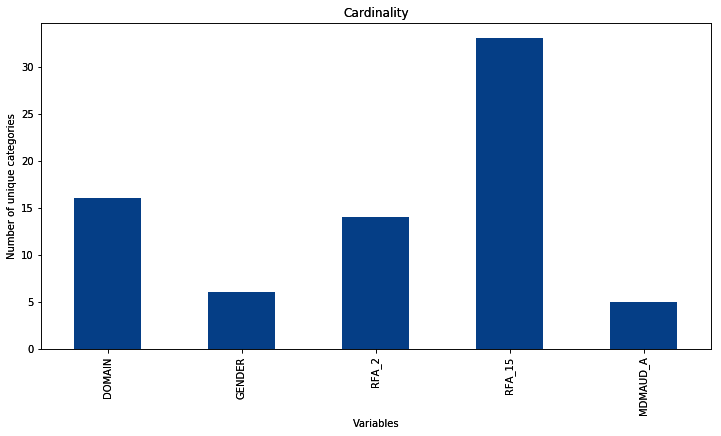We can change the figure size with the figsize argument and also add x and y labels and a title with plt.xlabel(), plt.ylabel(), and plt.title() to enhance the aesthetics of the plot.

# How it works...

In this recipe, we quantified and plotted the cardinality of the categorical variables of a publicly available dataset.

To load the categorical columns from the dataset, we captured the variable names in a list. Next, we used pandas read_csv() to load the data from a txt file onto a dataframe and passed the list with variable names to the usecols argument.

Many variables from the KDD-CUP-98 dataset contained empty strings which are, in essence, missing values. Thus, we replaced the empty strings with the NumPy representation of missing values, np.nan, by utilizing the pandas replace() method. With the head() method, we displayed the top five rows of the dataframe.

To quantify cardinality, we used the nunique() method from pandas, which finds and then counts the number of distinct values per variable. Next, we used the unique() method to output the distinct categories in the GENDER variable.

To plot the variable cardinality, we used pandas nunique(), followed by pandas plot.bar(), to make a bar plot with the variable cardinality, and added axis labels and a figure title by utilizing the Matplotlib xlabel(), ylabel(), and title() methods.

# There's more...

The nunique() method determines the number of unique values for categorical and numerical variables. In this recipe, we only used nunique() on categorical variables to explore the concept of cardinality. However, we could also use nunique() to evaluate numerical variables.

We can also evaluate the cardinality of a subset of the variables in a dataset by slicing the dataframe:

`data[['RFA_2', 'MDMAUD_A', 'RFA_2']].nunique()`

The following is the output of the preceding code:

```RFA_2       14
MDMAUD_A     5
RFA_2       14
dtype: int64```

In the preceding output, we can see the number of distinct values each of these variables can take.

# Pinpointing rare categories in categorical variables

Different labels appear in a variable with different frequencies. Some categories of a variable appear a lot, that is, they are very common among the observations, whereas other categories appear only in a few observations. In fact, categorical variables often contain a few dominant labels that account for the majority of the observations and a large number of labels that appear only seldom. Categories that appear in a tiny proportion of the observations are rare. Typically, we consider a label to be rare when it appears in less than 5% or 1% of the population. In this recipe, we will learn how to identify infrequent labels in a categorical variable.

To follow along with this recipe, download the Car Evaluation dataset from the UCI Machine Learning Repository by following the instructions in the Technical requirements section of this chapter.

# How to do it...

Let's begin by importing the necessary libraries and getting the data ready:

1. Import the required Python libraries:
`import pandas as pdimport matplotlib.pyplot as plt`
1. Let's load the Car Evaluation dataset, add the column names, and display the first five rows:
`data = pd.read_csv('car.data', header=None)data.columns = ['buying', 'maint', 'doors', 'persons', 'lug_boot', 'safety', 'class']data.head()`

We get the following output when the code is executed from a Jupyter Notebook: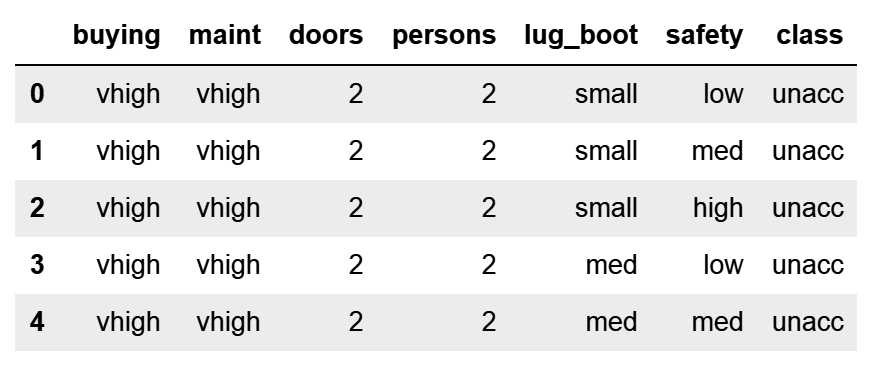By default, pandas read_csv() uses the first row of the data as the column names. If the column names are not part of the raw data, we need to specifically tell pandas not to assign the column names by adding the header = None argument.
1. Let's display the unique categories of the variable class:
`data['class'].unique()`

We can see the unique values of class in the following output:

`array(['unacc', 'acc', 'vgood', 'good'], dtype=object)`
1. Let's calculate the number of cars per category of the class variable and then divide them by the total number of cars in the dataset to obtain the percentage of cars per category. Then, we'll print the result:
`label_freq = data['class'].value_counts() / len(data)print(label_freq)`

The output of the preceding code block is a pandas Series, with the percentage of cars per category expressed as decimals:

```unacc    0.700231
acc      0.222222
good     0.039931
vgood    0.037616
Name: class, dtype: float64```
1. Let's make a bar plot showing the frequency of each category and highlight the 5% mark with a red line:
`fig = label_freq.sort_values(ascending=False).plot.bar()fig.axhline(y=0.05, color='red')fig.set_ylabel('percentage of cars within each category')fig.set_xlabel('Variable: class')fig.set_title('Identifying Rare Categories')plt.show()`

The following is the output of the preceding block code: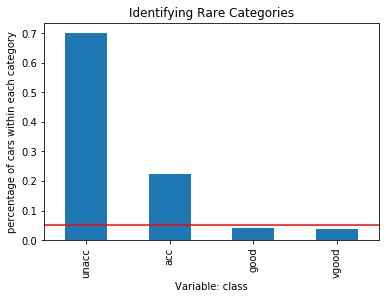The good and vgood categories are present in less than 5% of cars, as indicated by the red line in the preceding plot.

# How it works...

In this recipe, we quantified and plotted the percentage of observations per category, that is, the category frequency in a categorical variable of a publicly available dataset.

To load the data, we used pandas read_csv() and set the header argument to None, since the column names were not part of the raw data. Next, we added the column names manually by passing the variable names as a list to the columns attribute of the dataframe.

To determine the frequency of each category in the class variable, we counted the number of cars per category using pandas value_counts() and divided the result by the total cars in the dataset, which is determined with the Python built-in len method. Python's len method counted the number of rows in the dataframe. We captured the returned percentage of cars per category, expressed as decimals, in the label_freq variable.

To make a plot of the category frequency, we sorted the categories in label_freq from that of most cars to that of the fewest cars using the pandas sort_values() method. Next, we used plot.bar() to produce a bar plot. With axhline(), from Matplotlib, we added a horizontal red line at the height of 0.05 to indicate the 5% percentage limit, under which we considered a category as rare. We added x and y labels and a title with plt.xlabel(), plt.ylabel(), and plt.title() from Matplotlib.

# Identifying a linear relationship

Linear models assume that the independent variables, X, take a linear relationship with the dependent variable, Y. This relationship can be dictated by the following equation:Here, X specifies the independent variables and β are the coefficients that indicate a unit change in Y per unit change in X. Failure to meet this assumption may result in poor model performance.

Linear relationships can be evaluated by scatter plots and residual plots. Scatter plots output the relationship of the independent variable X and the target Y. Residuals are the difference between the linear estimation of Y using X and the real target:If the relationship is linear, the residuals should follow a normal distribution centered at zero, while the values should vary homogeneously along the values of the independent variable. In this recipe, we will evaluate the linear relationship using both scatter and residual plots in a toy dataset.

# How to do it...

Let's begin by importing the necessary libraries:

1. Import the required Python libraries and a linear regression class:
`import pandas as pdimport numpy as npimport matplotlib.pyplot as pltimport seaborn as snsfrom sklearn.linear_model import LinearRegression`

To proceed with this recipe, let's create a toy dataframe with an x variable that follows a normal distribution and shows a linear relationship with a y variable.

1. Create an x variable with 200 observations that are normally distributed:
`np.random.seed(29)x = np.random.randn(200)`
Setting the seed for reproducibility using np.random.seed() will help you get the outputs shown in this recipe.
1. Create a y variable that is linearly related to x with some added random noise:
`y = x * 10 + np.random.randn(200) * 2`
1. Create a dataframe with the x and y variables:
`data = pd.DataFrame([x, y]).Tdata.columns = ['x', 'y']`
1. Plot a scatter plot to visualize the linear relationship:
`sns.lmplot(x="x", y="y", data=data, order=1)plt.ylabel('Target')plt.xlabel('Independent variable')`

The preceding code results in the following output: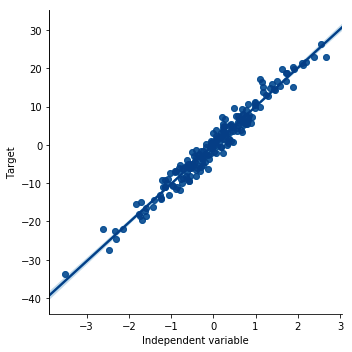To evaluate the linear relationship using residual plots, we need to carry out a few more steps.

1. Build a linear regression model between x and y:
`linreg = LinearRegression()linreg.fit(data['x'].to_frame(), data['y'])`
Scikit-learn predictor classes do not take pandas Series as arguments. Because data['x'] is a pandas Series, we need to convert it into a dataframe using to_frame().

Now, we need to calculate the residuals.

1. Make predictions of y using the fitted linear model:
`predictions = linreg.predict(data['x'].to_frame())`
1. Calculate the residuals, that is, the difference between the predictions and the real outcome, y:
`residuals = data['y'] - predictions`
1. Make a scatter plot of the independent variable x and the residuals:
`plt.scatter(y=residuals, x=data['x'])plt.ylabel('Residuals')plt.xlabel('Independent variable x')`

The output of the preceding code is as follows: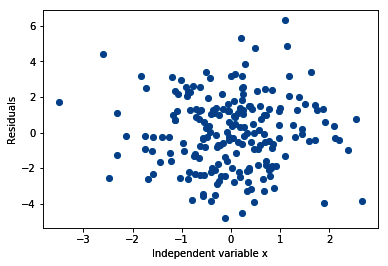1. Finally, let's evaluate the distribution of the residuals:
`sns.distplot(residuals, bins=30)plt.xlabel('Residuals')`

In the following output, we can see that the residuals are normally distributed and centered around zero: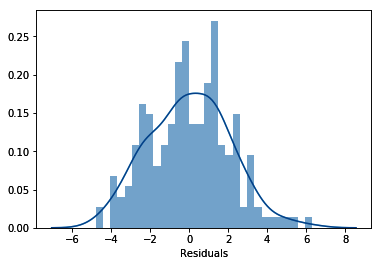Check the accompanying Jupyter Notebook for examples of scatter and residual plots in variables from a real dataset which can be found at https://github.com/PacktPublishing/Python-Feature-Engineering-Cookbook/blob/master/Chapter01/Recipe-5-Identifying-a-linear-relationship.ipynb.

# How it works...

In this recipe, we identified a linear relationship between an independent and a dependent variable using scatter and residual plots. To proceed with this recipe, we created a toy dataframe with an independent variable x that is normally distributed and linearly related to a dependent variable y. Next, we created a scatter plot between x and y, built a linear regression model between x and y, and obtained the predictions. Finally, we calculated the residuals and plotted the residuals versus the variable and the residuals histogram.

To generate the toy dataframe, we created an independent variable x that is normally distributed using NumPy's random.randn(), which extracts values at random from a normal distribution. Then, we created the dependent variable y by multiplying x 10 times and added random noise using NumPy's random.randn(). After, we captured x and y in a pandas dataframe using the pandas DataFrame() method and transposed it using the T method to return a 200 row x 2 column dataframe. We added the column names by passing them in a list to the columns dataframe attribute.

To create the scatter plot between x and y, we used the seaborn lmplot() method, which allows us to plot the data and fit and display a linear model on top of it. We specified the independent variable by setting x='x', the dependent variable by setting y='y', and the dataset by setting data=data. We created a model of order 1 that is a linear model, by setting the order argument to 1.

Seaborn lmplot() allows you to fit many polynomial models. You can indicate the order of the model by utilizing the order argument. In this recipe, we fit a linear model, so we indicated order=1

Next, we created a linear regression model between x and y using the LinearRegression() class from scikit-learn. We instantiated the model into a variable called linreg and then fitted the model with the fit() method with x and y as arguments. Because data['x'] was a pandas Series, we converted it into a dataframe with the to_frame() method. Next, we obtained the predictions of the linear model with the predict() method.

To make the residual plots, we calculated the residuals by subtracting the predictions from y. We evaluated the distribution of the residuals using seaborn's distplot(). Finally, we plotted the residuals against the values of x using Matplotlib scatter() and added the axis labels by utilizing Matplotlib's xlabel() and ylabel() methods.

# There's more...

In the GitHub repository of this book (https://github.com/PacktPublishing/Python-Feature-Engineering-Cookbook), there are additional demonstrations that use variables from a real dataset. In the Jupyter Notebook, you will find the example plots of variables that follow a linear relationship with the target, variables that are not linearly related.

# Identifying a normal distribution

Linear models assume that the independent variables are normally distributed. Failure to meet this assumption may produce algorithms that perform poorly. We can determine whether a variable is normally distributed with histograms and Q-Q plots. In a Q-Q plot, the quantiles of the independent variable are plotted against the expected quantiles of the normal distribution. If the variable is normally distributed, the dots in the Q-Q plot should fall along a 45 degree diagonal. In this recipe, we will learn how to evaluate normal distributions using histograms and Q-Q plots.

# How to do it...

Let's begin by importing the necessary libraries:

1. Import the required Python libraries and modules:
`import pandas as pdimport numpy as npimport matplotlib.pyplot as pltimport seaborn as snsimport scipy.stats as stats`

To proceed with this recipe, let's create a toy dataframe with a single variable, x, that follows a normal distribution.

1. Create a variable, x, with 200 observations that are normally distributed:
`np.random.seed(29)x = np.random.randn(200)`
Setting the seed for reproducibility using np.random.seed() will help you get the outputs shown in this recipe.
1. Create a dataframe with the x variable:
`data = pd.DataFrame([x]).Tdata.columns = ['x']`
1. Make a histogram and a density plot of the variable distribution:
`sns.distplot(data['x'], bins=30)`

The output of the preceding code is as follows: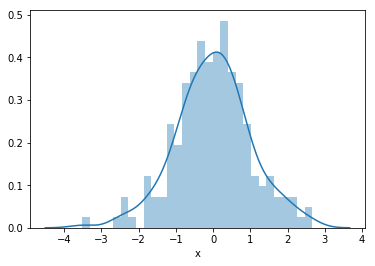We can also create a histogram using the pandas hist() method, that is, data['x'].hist(bins=30).
1. Create and display a Q-Q plot to assess a normal distribution:
`stats.probplot(data['x'], dist="norm", plot=plt)plt.show()`

The output of the preceding code is as follows: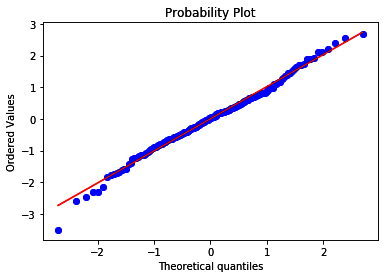Since the variable is normally distributed, its values follow the theoretical quantiles and thus lie along the 45-degree diagonal.

# How it works...

In this recipe, we determined whether a variable is normally distributed with a histogram and a Q-Q plot. To do so, we created a toy dataframe with a single independent variable, x, that is normally distributed, and then created a histogram and a Q-Q plot.

For the toy dataframe, we created a normally distributed variable, x, using the NumPy random.randn() method, which extracted 200 random values from a normal distribution. Next, we captured x in a dataframe using the pandas DataFrame() method and transposed it using the T method to return a 200 row x 1 column dataframe. Finally, we added the column name as a list to the dataframe's columns attribute.

To display the variable distribution as a histogram and density plot, we used seaborn's distplot() method. By setting the bins argument to 30, we created 30 contiguous intervals for the histogram. To create the Q-Q plot, we used stats.probplot() from SciPy, which generated a plot of the quantiles for our x variable in the y-axis versus the quantiles of a theoretical normal distribution, which we indicated by setting the dist argument to norm, in the x-axis. We used Matplotlib to display the plot by setting the plot argument to pltSince x was normally distributed, its quantiles followed the quantiles of the theoretical distribution, so that the dots of the variable values fell along the 45-degree line.

# There's more...

For more details about seaborn's distplot or SciPy's Q-Q plots, take a look at the following links:

# Distinguishing variable distribution

A probability distribution is a function that describes the likelihood of obtaining the possible values of a variable. There are many well-described variable distributions, such as the normal, binomial, or Poisson distributions. Some machine learning algorithms assume that the independent variables are normally distributed. Other models make no assumptions about the distribution of the variables, but a better spread of these values may improve their performance. In this recipe, we will learn how to create plots to distinguish the variable distributions in the entire dataset by using the Boston House Prices dataset from scikit-learn.

# How to do it...

Let's begin by importing the necessary libraries:

1. Import the required Python libraries and modules:
`import pandas as pdimport matplotlib.pyplot as plt`
1. Load the Boston House Prices dataset from scikit-learn:
`from sklearn.datasets import load_bostonboston_dataset = load_boston()boston = pd.DataFrame(boston_dataset.data,         columns=boston_dataset.feature_names)`
1. Visualize the variable distribution with histograms:
`boston.hist(bins=30, figsize=(12,12), density=True)plt.show()`

The output of the preceding code is shown in the following screenshot: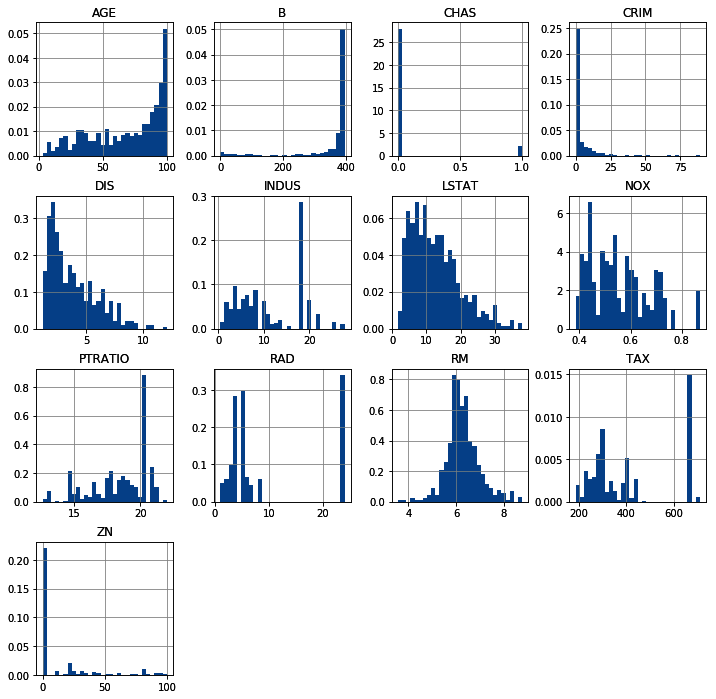Most of the numerical variables in the dataset are skewed.

# How it works...

In this recipe, we used pandas hist() to plot the distribution of all the numerical variables in the Boston House Prices dataset from scikit-learn. To load the data, we imported the dataset from scikit-learn datasets and then used load_boston() to load the data. Next, we captured the data into a dataframe using pandas DataFrame(), indicating that the data is stored in the data attribute and the variable names in the feature_names attribute.

To display the histograms of all the numerical variables, we used pandas hist(), which calls matplotlib.pyplot.hist() on each variable in the dataframe, resulting in one histogram per variable. We indicated the number of intervals for the histograms using the bins argument, adjusted the figure size with figsize, and normalized the histogram by setting density to TrueIf the histogram is normalized, the sum of the area under the curve is 1.

# Highlighting outliers

An outlier is a data point that is significantly different from the remaining data. On occasions, outliers are very informative; for example, when looking for credit card transactions, an outlier may be an indication of fraud. In other cases, outliers are rare observations that do not add any additional information. These cases may also affect the performance of some machine learning models.

"An outlier is an observation which deviates so much from the other observations as to arouse suspicions that it was generated by a different mechanism." [D. Hawkins. Identification of Outliers, Chapman and Hall, 1980.]

In this recipe, we will learn how to identify outliers using boxplots and the inter-quartile range (IQR) proximity rule. According to the IQR proximity rule, a value is an outlier if it falls outside these boundaries:

Upper boundary = 75th quantile + (IQR * 1.5)

Lower boundary = 25th quantile - (IQR * 1.5)

Here, IQR is given by the following equation:

IQR = 75th quantile - 25th quantile

Typically, we calculate the IQR proximity rule boundaries by multiplying the IQR by 1.5. However, it is also common practice to find extreme values by multiplying the IQR by 3.

# How to do it...

Let's begin by importing the necessary libraries and preparing the dataset:

1. Import the required Python libraries and the dataset:
`import pandas as pdimport numpy as npimport matplotlib.pyplot as pltimport seaborn as snsfrom sklearn.datasets import load_boston`
1. Load the Boston House Prices dataset from scikit-learn and retain three of its variables in a dataframe:
`boston_dataset = load_boston()boston = pd.DataFrame(boston_dataset.data, columns=boston_dataset.feature_names)[['RM', 'LSTAT', 'CRIM']]`
1. Make a boxplot for the RM variable:
`sns.boxplot(y=boston['RM'])plt.title('Boxplot')`

The output of the preceding code is as follows: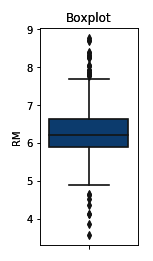We can change the final size of the plot using the figure() method from Matplotlib. We need to call this command before making the plot with seaborn:
plt.figure(figsize=(3,6))
sns.boxplot(y=boston['RM'])
plt.title('Boxplot')

To find the outliers in a variable, we need to find the distribution boundaries according to the IQR proximity rule, which we discussed in the Getting ready section of this recipe.

1. Create a function that takes a dataframe, a variable name, and the factor to use in the IQR calculation and returns the IQR proximity rule boundaries:
`def find_boundaries(df, variable, distance):    IQR = df[variable].quantile(0.75) - df[variable].quantile(0.25)    lower_boundary = df[variable].quantile(0.25) - (IQR * distance)    upper_boundary = df[variable].quantile(0.75) + (IQR * distance)    return upper_boundary, lower_boundary`
1. Calculate and then display the IQR proximity rule boundaries for the RM variable:
`upper_boundary, lower_boundary = find_boundaries(boston, 'RM', 1.5)upper_boundary, lower_boundary`

The find_boundaries() function returns the values above and below which we can consider a value to be an outlier, as shown here:

`(7.730499999999999, 4.778500000000001)`
If you want to find very extreme values, you can use 3 as the distance of find_boundaries() instead of 1.5.

Now, we need to find the outliers in the dataframe.

1. Create a boolean vector to flag observations outside the boundaries we determined in step 5:
`outliers = np.where(boston['RM'] > upper_boundary, True,            np.where(boston['RM'] < lower_boundary, True, False))`
1. Create a new dataframe with the outlier values and then display the top five rows:
`outliers_df = boston.loc[outliers, 'RM']outliers_df.head()`

We can see the top five outliers in the RM variable in the following output:

```97     8.069
98     7.820
162    7.802
163    8.375
166    7.929
Name: RM, dtype: float64```

To remove the outliers from the dataset, execute boston.loc[~outliers, 'RM'].

# How it works...

In this recipe, we identified outliers in the numerical variables of the Boston House Prices dataset from scikit-learn using boxplots and the IQR proximity rule. To proceed with this recipe, we loaded the dataset from scikit-learn and created a boxplot for one of its numerical variables as an example. Next, we created a function to identify the boundaries using the IQR proximity rule and used the function to determine the boundaries of the numerical RM variable. Finally, we identified the values of RM that were higher or lower than those boundaries, that is, the outliers.

To load the data, we imported the dataset from sklearn.datasets and used load_boston(). Next, we captured the data in a dataframe using pandas DataFrame(), indicating that the data was stored in the data attribute and that the variable names were stored in the feature_names attribute. To retain only the RM, LSTAT, and CRIM variables, we passed the column names in double brackets [[]] at the back of pandas DataFrame().

To display the boxplot, we used seaborn's boxplot() method and passed the pandas Series with the RM variable as an argument. In the boxplot displayed after step 3, the IQR is delimited by the rectangle, and the upper and lower boundaries corresponding to either, the 75th quantile plus 1.5 times the IQR, or the 25th quantile minus 1.5 times the IQR. This is indicated by the whiskers. The outliers are the asterisks lying outside the whiskers.

To identify those outliers in our dataframe, in step 4, we created a function to find the boundaries according to the IQR proximity rule. The function took the dataframe and the variable as arguments and calculated the IQR and the boundaries using the formula described in the Getting ready section of this recipe. With the pandas quantile() method, we calculated the values for the 25th (0.25) and 75th quantiles (0.75). The function returned the upper and lower boundaries for the RM variable.

To find the outliers of RM, we used NumPy's where() method, which produced a boolean vector with True if the value was an outlier. Briefly, where() scanned the rows of the RM variable, and if the value was bigger than the upper boundary, it assigned True, whereas if the value was smaller, the second where() nested inside the first one and checked whether the value was smaller than the lower boundary, in which case it also assigned True, otherwise False. Finally, we used the loc[] method from pandas to capture only those values in the RM variable that were outliers in a new dataframe.

# Comparing feature magnitude

Many machine learning algorithms are sensitive to the scale of the features. For example, the coefficients of linear models are directly informed by the scale of the feature. In addition, features with bigger value ranges tend to dominate over features with smaller ranges. Having features within a similar scale also helps algorithms converge faster, thus improving performance and training times. In this recipe, we will explore and compare feature magnitude by looking at statistical parameters such as the mean, median, standard deviation, and maximum and minimum values by leveraging the power of pandas.

For this recipe, you need to be familiar with common statistical parameters such as mean, quantiles, maximum and minimum values, and standard deviation. We will use the Boston House Prices dataset included in scikit-learn to do this.

# How to do it...

1. Import the required Python libraries and classes:
`import pandas as pdfrom sklearn.datasets import load_boston`
1. Load the Boston House Prices dataset from scikit-learn into a dataframe:
`boston_dataset = load_boston()data = pd.DataFrame(boston_dataset.data, columns=boston_dataset.feature_names)`
1. Print the main statistics for each variable in the dataset, that is, the mean, count, standard deviation, median, quantiles, and minimum and maximum values:
`data.describe()`

The following is the output of the preceding code when we run it from a Jupyter Notebook: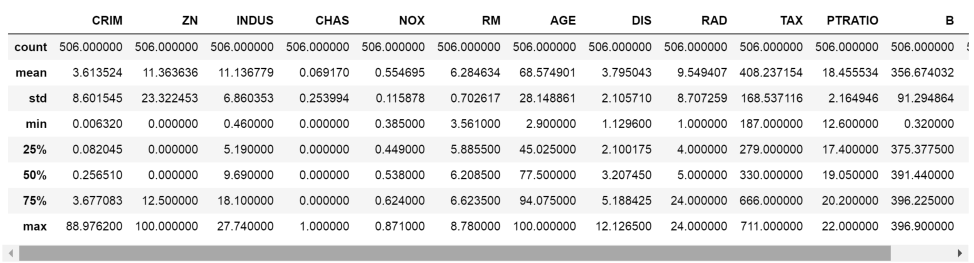1. Calculate the value range of each variable, that is, the difference between the maximum and minimum value:
`data.max() - data.min()`

The following output shows the value ranges of the different variables:

```CRIM        88.96988
ZN         100.00000
INDUS       27.28000
CHAS         1.00000
NOX          0.48600
RM           5.21900
AGE         97.10000
DIS         10.99690
TAX        524.00000
PTRATIO      9.40000
B          396.58000
LSTAT       36.24000
dtype: float64```

The value ranges of the variables are quite different.

# How it works...

In this recipe, we used the describe() method from pandas to return the main statistical parameters of a distribution, namely, the mean, standard deviation, minimum and maximum values, 25th, 50th, and 75th quantiles, and the number of observations (count).

We can also calculate these parameters individually using the pandas mean(), count(), min(), max(), std(), and quantile() methods.

Finally, we calculated the value range by subtracting the minimum from the maximum value in each variable using the pandas max() and min() methods.